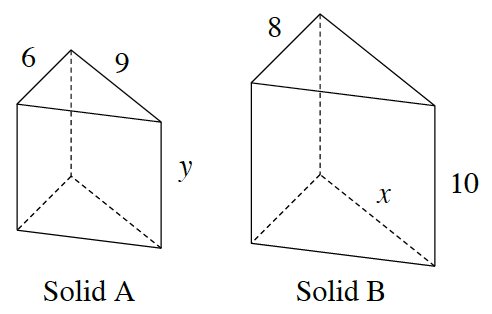Home > CCG > Chapter 11 > Lesson 11.1.3 > Problem11-43

11-43.Assume that the prisms at right are similar.

1. Solve for $x$ and $y$.

Remember that the ratios of corresponding sides are equal if two triangles are similar.

1. What is the ratio of the corresponding sides of Solid $B$ to Solid $A$?

$\frac{4}{3}$

2. If the base area of Solid $A$ is $27$ square units, find the base area of Solid $B$.

$\text{Base area of solid}=(27)\left(\frac{4}{3}\right)^2$